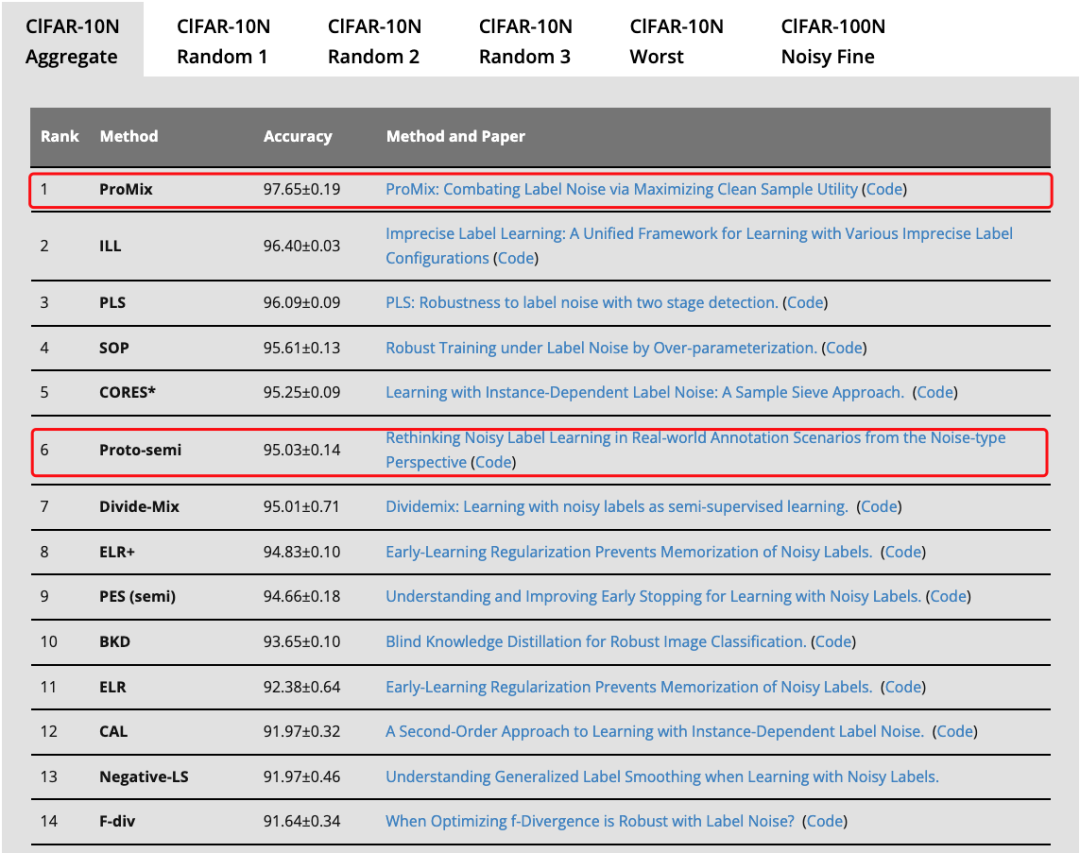# id3算法生成决策树的过程（附实现代码）

ID3算法是一种用于生成决策树的经典算法，它是在1986年由Ross Quinlan提出的。该算法通过计算每个特征的信息增益来确定最佳特征，然后将该特征用作分裂节点。ID3算法被广泛应用于机器学习和数据挖掘领域，特别是在分类任务中。

ID3算法的基本思路是在每个节点上选择最佳特征，将数据集分成更小的子集，然后递归地对每个子集应用相同的过程，直到达到终止条件。在分类问题中，终止条件通常是所有实例属于同一类别或没有更多特征可供分裂。在回归问题中，终止条件通常是达到一定的误差或深度限制。

### ID3算法生成决策树的过程

1.选取最佳特征

IG(D,F)=H(D)-\sum_{v\in Values(F)}\frac{|D_v|}{|D|}H(D_v)

2.将数据集分成子集

3.递归生成子树

4.构建决策树

### ID3算法的Python实现代码

import mathclass DecisionTree:    def __init__(self):        self.tree = {}    def fit(self, X, y):        self.tree = self._build_tree(X, y)    def predict(self, X):        y_pred = []        for i in range(len(X)):            node = self.tree            while isinstance(node, dict):                feature = list(node.keys())                value = X[i][feature]                node = node[feature][value]            y_pred.append(node)        return y_pred    def _entropy(self, y):        n = len(y)        counts = {}        for value in y:            counts[value] = counts.get(value, 0) + 1        entropy = 0        for count in counts.values():            p = count / n            entropy -= p * math.log2(p)        return entropy    def _information_gain(self, X, y, feature):        n = len(y)        values = set([x[feature] for x in X])        entropy = 0        for value in values:            subset_x = [x forx in X if x[feature] == value]            subset_y = [y[i] for i in range(len(y)) if X[i][feature] == value]            entropy += len(subset_y) / n * self._entropy(subset_y)        information_gain = self._entropy(y) - entropy        return information_gain    def _majority_vote(self, y):        counts = {}        for value in y:            counts[value] = counts.get(value, 0) + 1        majority = max(counts, key=counts.get)        return majority    def _build_tree(self, X, y):        if len(set(y)) == 1:            return y        if len(X) == 0:            return self._majority_vote(y)        best_feature = max(range(len(X)), key=lambda i: self._information_gain(X, y, i))        tree = {best_feature: {}}        values = set([x[best_feature] for x in X])        for value in values:            subset_x = [x for x in X if x[best_feature] == value]            subset_y = [y[i] for i in range(len(y)) if X[i][best_feature] == value]            subtree = self._build_tree(subset_x, subset_y)            tree[best_feature][value] = subtree        return tree

2023-09-27 10:07:19

2023-09-27 10:02:13

2023-09-26 09:57:222023-09-22 09:59:122023-09-21 10:11:30

2023-09-21 09:55:18

Attention机制是一种处理序列数据的重要算法，其核心思想是为每个输入序列中的元素分配一个权重，以便在计算输出时考虑这些元素的相对重要性。这种机制已经被广泛应用于自然语言处理、图像处理以及其他领域。下面将介绍几种基于attention机制的算法及其应用。
2023-09-20 10:08:33
Attention模型详解
Attention模型是深度学习中的一种重要模型，它能够帮助模型处理序列数据，从而在机器翻译、语音识别、图像处理等领域中取得了很好的效果。本文将详细介绍Attention模型的原理、应用和发展。
2023-09-20 10:04:47
HITS算法（计算网页权威性和相关性）
HITS算法是一种用于计算网页权威性和主题相关性的算法，是搜索引擎排名算法中的一种。HITS算法通过分析页面之间的链接关系，将页面分为两类：权威页面和枢纽页面。权威页面是被其他页面高度引用的页面，而枢纽页面是链接到权威页面的页面。HITS算法通过递归地计算页面的权威值和枢纽值，来确定网页的排名。
2023-09-19 10:11:23
Alpha-beta剪枝算法
Alpha-beta剪枝算法是一种用于优化搜索树的算法，通常用于博弈论和其他搜索问题，可以大大减少搜索的时间和空间复杂度。该算法是对极小极大算法的扩展，它利用了剪枝技术来减少搜索树中的节点数，以便在更短的时间内找到最优解。
2023-09-18 10:09:26